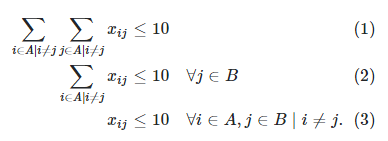•Gurobi Staff

The addVar method adds a single variable while the addVars method adds multiple variables at once. The addVars method is a convenience method, since you can get the exact same result by using a $$\texttt{for}$$-loop and the addVar method.

import gurobipy as gpm = gp.Model()x = m.addVars(3, name="x")m.update()print(x)

generates 3 variables named $$\texttt{"x","x","x"}$$, which can be accessed via $$\texttt{x,x,x}$$.

The equivalent code using addVar would be

import gurobipy as gpm = gp.Model()x = {}for i in range(3):    x[i] = m.addVar(name="x[%d]"%i)m.update()print(x)

The same difference holds for addConstr and addConstrs. There should be no significant performance difference between the two respective methods.

Usually, adding sets of optimization variables through addVars results in a more concise code.

For constraints, it is recommended to use addConstr if the generated constraints are very complex, i.e., when many $$\texttt{for}$$-loops and $$\texttt{if}$$-clauses are needed to iterate through the indices. Otherwise, using addConstrs to add a set of constraints simultaneously results in a more concise code.

You could browse through the Python examples to get a feeling when to use which method. Our webinar on gurobipy should also bring some clarity.

Best regards,
Jaromił

•Thanks for a great explanation!

•